# PowerApps - Sort, SortByColumns and SortOrder functions

In this article, we are going to go through two different Sorting functions provided by PowerApps. Sort and SortByColumns. Let’s understand in detail, how and where you can use each one of them, what are the pros and cons of each over the other using some examples.

Note:

• When you want to sort on a set of record/table /collection you can use either Sort or SortByColumns function in PowerApps.
• PowerApps sorting can be done either by a column of a table or using a formula.
• The output of the formula must be either a Number or a String or a Boolean value. It cannot be a RowItem/Record/Table.

## PowerApps Sort function Syntax

Sort(Table, Formula, SortOrder)

• Table: This is required. Pass the table or collection or data source to sort.
• Formula: This is required
• You can execute a formula on the table mentioned in the first parameter.
• The result which comes after applying the formula will be used to sort the table.
• SortOrder: This is optional. You can specify SortOrder.Descending when you want to sort the table in descending order. If you don’t specify anything in this parameter, it will consider SortOrder.Ascending as it is the default sort order.

## PowerApps SortByColumns function Syntax

SortByColumns(Table, ColumnName1, SortOrder1, ColumnName2, SortOrder2, …, …)

• Table: This is required. Pass the table or collection or data source to sort.
• ColumnName(s): This is required.
• You can specify the name of the column from the table or collection.
• Here, you need to specify the internal name of the column in quotes
• You can specify multiple columns over here.
• SortOrder: This is optional.
• You can specify SortOrder.Descending when you want to sort the table in descending order.
• If you don’t specify anything in this parameter, it will consider SortOrder.Ascending as it is the default sort order.
• For each column name you specify in the Second parameter, you need to specify the sort order separately as mentioned in the syntax.

## PowerApps Sort and SortByColumns Functions Examples

We will use the below table for our examples.

Table Name: Weathers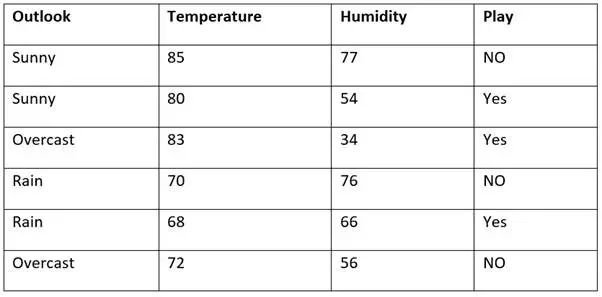## PowerApps sort by the number field

• Here we are going to sort a table based on a Numeric column.
• Weather is the table name and Humidity is the Numeric field
• The first parameter is the database/table name, the second parameter is the column name and the third parameter is Order (Ascending or Descending). If you don’t specify anything here, it will consider Ascending(default).
• In the example below, you can observe that the Sort function has the Display name of the column without quotes and the SortByColumns function has the internal name of the column with quotes.

// Syntax of Sort and SortByColumns function.

Sort(Weathers, Humidity)

// OR

SortByColumns(Weathers,"data_humidity")

Output: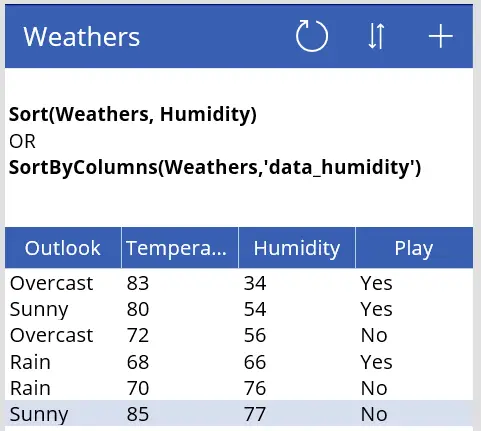• Here, we are going to sort a table based on a DateTime column in the table
• We can apply sorting on the database/table just like we did for the Numeric field.
• Weather is the table name and DateDisplayed is the DateTime field
• Be extra careful when you display the DateTime column and ensure to convert into the format you desire.
• DateTime Format
• I have applied the dd/mm/yyyy format in the below table for the column value display.
• Text(ThisItem.DateDisplayed,"dd/mm/yyyy")
• Here, DateDisplayed is already a DateTime column. If it is not, then you can convert first into DateTimeValue and then apply the format as mentioned below.
Text(DateTimeValue(ThisItem.DateDisplayed), "dd/mm/yyyy")

Sort(Weathers, DateDisplayed)

// OR

SortByColumns(Weathers,"data_datedisplayed")

Output: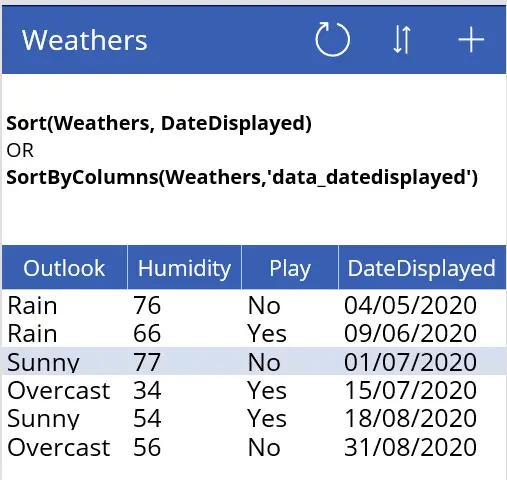• Here, we are going to sort the table using multiple columns.
• Here, we will first sort by Humidity column and then by temperature column.
• The syntax for Sort and SortByColumns is totally different in this case.
• Explanation: Here, the first sort is applied to the Weather table by the Humidity column. Then on the resulting data, the second sorting is applied.
• In this example, the second sorting is descending.

Sort(Sort(Weathers,Humidity,Ascending),Temperature,Descending)

// OR

SortByColumns(Weathers,"data_humidity",Ascending,"data_temperature",Descending)

Output: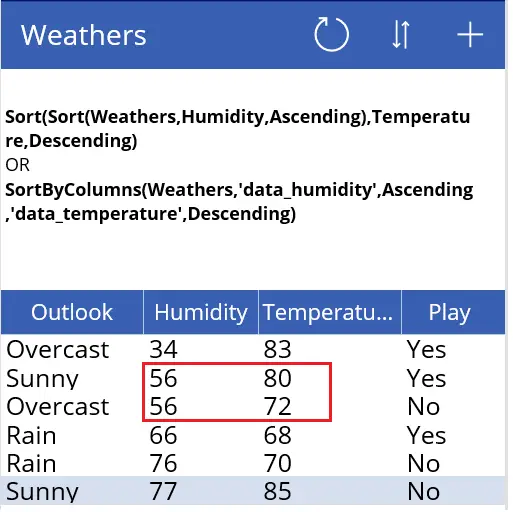## PowerApps sort dropdown alphabetically

• Here, we will see how to sort dropdown list values.
• There are two cases here.

1. Specify hardcoded values in Dropdown list and sort them.

Example:

Sort(["Humidity","Temperature","Outlook","Play"], Value, Ascending)

You can see in the output that values are sorted in ascending order.

Output: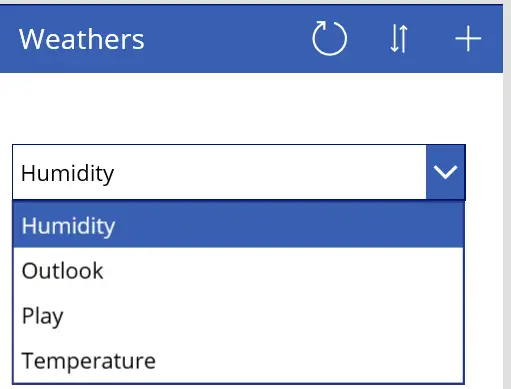2. Specify column values from a table in Dropdown list and sort them.

In this example, we are going to select distinct values from a column and SORT them.

• First, we are going to
• Select Distinct Temperature values from Weathers table
• Then on the Resulting table, we are going to apply the DESCENDING sort.

Example: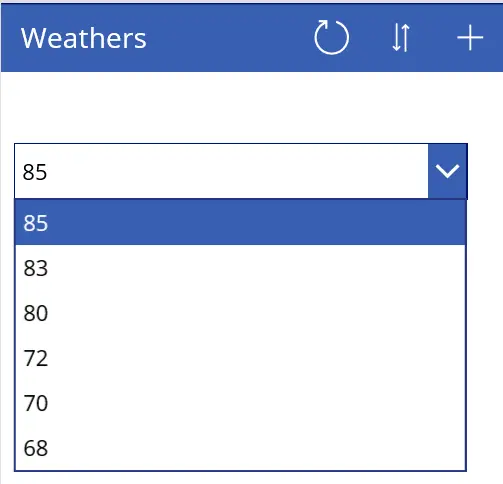Sort(Distinct(Weathers, Temperature), Result, Descending)

Let us know if you have any difficulties using the Sort or SortByColumns function, we will try to cover them here.

Page URL:

Name:

Email:

Suggestion: